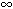DOC HOME SITE MAP MAN PAGES GNU INFO SEARCH PRINT BOOK

# remainder(3M)

remainder, remquo -- remainder functions

## Synopsis

```   cc [flag ...] file ...  -lm [library ...]

#include <math.h>

double remainder(double x, double y);

float remainderf(float x, float y);

long double remainderl(long double x, long double y);

double remquo(double x, double y, int *quo);

float remquof(float x, float y, int *quo);

long double remquol(long double x, long double y, int *quo);
```

## Description

remainder, remainderf and remainderl return the floating point remainder of the division of x by y. More precisely, it returns the value r = x - yn, where n is the integer nearest the exact value x/y. Whenever |n - x/y| = ½, then n is even.

remquo, remquof and remquol return the floating point remainder of the division of x by y. In the object pointed to by quo they store a value whose sign is the sign of x/y and whose magnitude is congruent modulo 2^n to the magnitude of the integral quotient of x/y, where n is an implementation-defined integer greater than or equal to 3.

NOTE: In UnixWare n is equal to 3.

### Errors

If x is not a NaN and y is ±0 remainder and remquo (and their floating and long double equivalents) return a NaN, raise an invalid operation exception and set errno to EDOM.

If x is ±and y is is not a NaN remainder and remquo (and their floating and long double equivalents) return a NaN, raise an invalid operation exception and set errno to EDOM.

On systems that support IEEE NaN, if the argument to any of these functions is a quiet NaN, that value is returned. If the argument is a signaling NaN, a quiet NaN is returned and the invalid operation exception is raised. In either case, errno is set to EDOM.

## References

cc(1), matherr(3M),